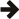#Boost C++ Libraries

...one of the most highly regarded and expertly designed C++ library projects in the world.## Overview

Boost.Integer provides integer type support, particularly helpful in generic programming. It provides standard C99 integer types, such as might be found in <stdint.h>, without requiring that header. It provides the means to select an integer type based upon its properties, like the number of bits or the maximum supported value, as well as compile-time bit mask selection. There is a derivative of std::numeric_limits that provides integral constant expressions for `min` and `max`. Finally, it provides two compile-time algorithms: determining the highest power of two in a compile-time value; and computing min and max of constant expressions.

Component

Purpose

Forward Declarations.

`<boost/integer_fwd.hpp>`

Forward declarations of classes and class templates - for use when just the name of a class is needed.

`<boost/cstdint.hpp>`

Provides typedef's based on the 1999 C Standard header `<stdint.h>`, wrapped in namespace boost. This implementation may #include the compiler supplied `<stdint.h>`, if present.

`<boost/integer_traits.hpp>`

Class template `boost::integer_traits`, derives from `std::numeric_limits` and adds `const_min` and `const_max` members.

`<boost/integer.hpp>`

Templates for integer type selection based on properties such as maximum value or number of bits: Use to select the type of an integer when some property such as maximum value or number of bits is known. Useful for generic programming.

`<boost/integer/integer_mask.hpp>`

Templates for the selection of integer masks, single or lowest group, based on the number of bits: Use to select a particular mask when the bit position(s) are based on a compile-time variable. Useful for generic programming.

`<boost/integer/static_log2.hpp>`

Template for finding the highest power of two in a number: Use to find the bit-size/range based on a maximum value. Useful for generic programming.

`<boost/integer/static_min_max.hpp>`

Templates for finding the extrema of two numbers: Use to find a bound based on a minimum or maximum value. Useful for generic programming.

 Last revised: July 08, 2010 at 20:45:20 GMT